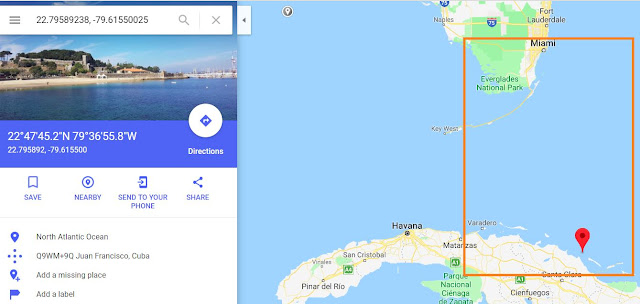## Monday, February 4, 2019

### twittter location clustering based on tweets (Spark Mllib)

1)  Create a directory for twitter streams
` cd /usr/lib/spark `
` sudo mkdir tweets `
``` cd tweetscd
sudo mkdir data ```
` sudo mkdir training`
` sudo chmod  777 /usr/lib/spark/tweets/ `

These are the two folders which we would be using in this project
data :Would contain the master of the csv files which we would pretend coming from a training source.
training :  Source to train our machine learning algorithm

```sudo wget https://raw.githubusercontent.com/chimpler/tweet-heatmap/master/tweets_tech.csv
```
Every record in this contains longitude and latitude position and the third column is the actual tweet

3) split the files
```sudo split tweets_tech.csv
```

4) Move all the  split files to data directory
```sudo mv /usr/lib/spark/tweets/x* /usr/lib/spark/tweets/data/
```

5) Create a spark streaming file
```sudo vi streaming_ml.py
```

```import sys

import sched, time

from pyspark import SparkContext
from pyspark.streaming import StreamingContext

from pyspark.mllib.linalg import Vectors
from pyspark.mllib.clustering import StreamingKMeans

if __name__ == "__main__":

sc = SparkContext(appName="StreamingKMeansClustering")
ssc = StreamingContext(sc, 10)

ssc.checkpoint("file:///tmp/spark")

def parseTrainingData(line):
cells = line.split(",")
return Vectors.dense([float(cells), float(cells)])

.map(parseTrainingData)

trainingStream.pprint();

model = StreamingKMeans(k=2, decayFactor=1.0).setRandomCenters(2, 1.0, 0)

print("Initial centers: " + str(model.latestModel().centers))

model.trainOn(trainingStream)

ssc.start()

s = sched.scheduler(time.time, time.sleep)
def print_cluster_centers(sc, model):
print("Cluster centers: " + str(model.latestModel().centers))
s.enter(10, 1, print_cluster_centers, (sc, model))

s.enter(10, 1, print_cluster_centers, (s, model))
s.run()

ssc.awaitTermination()
```

6) submit spark job
```spark-submit streaming_ml.py
```

Job job would start listening to the streaming cluster

****
Note:By default spark-submit might not work and would throw exception as shown below

"ImportError: No module named numpy".
Solution : Install pip and then numpy

`1) #CD to pyspark directory. You would find the path where error is thrown. `
```  #The path shown below is for my sandbox
/usr/hdp/current/spark-client/python/lib/pyspark
2) Install pip
yum install python-pip
3) install numpy
pip install numpy
4) unpgrade pip (This step might not be needed).
```

Spark streaming would be listening for the text stream as shown below.

7) Move data file from data folder to training folder
```sudo mv /usr/lib/spark/tweets/data/xaa /usr/lib/spark/twee
ts/training/
```

8) Before moving the file we would see the initial centers

Note:
1) While streaming any existing files in the directory would be ignored.only new files would be considered when we set the decay factor = 0.It would consider previous data when decay factor=1
2) While the new files arrives the cluster centers would be updated .The K means algorithm has calculated the cluster centers for where the location tweets are concentrated

9) Initial locations before the first file is moved to the training folder
[ 1.76405235 0.40015721 ]
[ 0.97873798 0.40015721 ]

DECAY FACTOR =1
When decay factor is set to 1, it would consider all the data from initial to the resent data.

We can see these locations using google maps as shown below

When we zoom out, the locations are as shown below. What we see over here is the tweet location is concentrated near Nigeria

10) Move the first file to the training folder.
```cp /usr/lib/spark/tweets/data/xaa /usr/lib/spark/tweets/training/
```

Co-ordinates for the 1st cluster
[22.79589238, -79.61550025]
Location after the first file is moved. It is somewhere between Florida and cuba for the cluster             centerCo-ordinates for the 2nd cluster
[10.03386625, 103.83779637]

11) Now move the 2nd file to the training folder.
The co-ordinates would change as shown below.
```cp /usr/lib/spark/tweets/data/xab /usr/lib/spark/tweets/training/
```

Finally after training with all the clusters we get the clustered center as shown below.
As location moved north cluster center also keep moving as shown below.

DECAY FACTOR =0
When we set the decay factor to 0.0 It would only consider the recent batch of data.

12) Delete all the files from the data directory.
`rm -r x*`

13)create a new streaming_ml.py file with decay factor as 0
14) Repeat step 10 and 11 and now we see that co-ordinates would move very far north as shown below

1.